# GSEB Solutions Class 11 Maths Chapter 4 Principle of Mathematical Induction Ex 4.1

Gujarat Board GSEB Textbook Solutions Class 11 Maths Chapter 4 Principle of Mathematical Induction Ex 4.1 Textbook Questions and Answers.

## Gujarat Board Textbook Solutions Class 11 Maths Chapter 4 Principle of Mathematical Induction Ex 4.1

Prove the following by using the principle of mathematical induction for all n ∈ N?
1. 1 + 3 + 32 + … + 3n-1 = $$\frac{3^{n}-1}{2}$$.
2. 13 + 23 + 33 + ………………. + n3 = ($$\frac{n(n+1)}{2}$$2.
3. 1 + $$\frac{1}{1+2}$$ + $$\frac{1}{1+2+3}$$ + ………….. + $$\frac{1}{1+2+3+………..+n}$$ = $$\frac{2n}{n+1}$$.
4. 1.2.3 + 2.3.4 + 3.4.5 + … + n(n + 1)(n + 2)
= $$\frac{n(n + 1)(n + 2)(n + 3)}{4}$$.
5. 1.3 + 2.32 + 3.32 + ……………. + n.3n = $$\frac{(2 n-1) \cdot 3^{n+1}+3}{4}$$
6. 1.2 + 2.3 + 3.4 + ……………… + n(n + 1) = $$\frac{n(n + 1)(n + 2)}{3}$$.
7. 1.3 + 3.5 + 5.7 + …………….. + (2n – 1)(2n + 1) = $$\frac{n\left(4 n^{2}+6 n-1\right)}{3}$$.
8. 1.2 + 2.22 + 3.22 + …………….. + n.2n = (n – 1).2n+1 + 2.
9. $$\frac{1}{2}$$ + $$\frac{1}{4}$$ + $$\frac{1}{8}$$ + ……………. + $$\frac{1}{2^{n}}$$ = 1 – $$\frac{1}{2^{n}}$$.
10. $$\frac{1}{2.5}$$ + $$\frac{1}{5.8}$$ + $$\frac{1}{8.11}$$ + ………………. + $$\frac{1}{(3n – 1)(3n + 2)}$$ = $$\frac{n}{6n + 4}$$.
11. $$\frac{1}{1.2.3}$$ + $$\frac{1}{2.3.4}$$ + $$\frac{1}{3.4.5}$$ + …………….. + $$\frac{1}{n(n + 1)(n + 2)}$$ = $$\frac{n(n + 3)}{4(n + 1)(n + 2)}$$.
12. a + ar + ar2 + …………… + arn-1 = $$\frac{a\left(1-r^{n}\right)}{1-r}$$.
13. (1 + $$\frac{3}{1}$$) (1 + $$\frac{5}{4}$$) (1 + $$\frac{7}{9}$$) ……………. (1 + $$\frac{2 n+1}{n^{2}}$$) = (n + 1)2
14. (1 + $$\frac{1}{1}$$) (1 + $$\frac{1}{2}$$) (1 + $$\frac{1}{3}$$) …………….. (1 + $$\frac{1}{n}$$) = n + 1.
15. 12 + 32 + 52 + ………….. + (2n – 1)2 = $$\frac{n(2n – 1)(2n + 1)}{3}$$.
16. $$\frac{1}{1.4}$$ + $$\frac{1}{4.7}$$ + $$\frac{1}{7.10}$$ + …………….. + $$\frac{1}{(3n – 2)(3n + 1)}$$ = $$\frac{n}{3n+1}$$.
17. $$\frac{1}{3.5}$$ + $$\frac{1}{5.7}$$ + $$\frac{1}{7.9}$$ + …………… + $$\frac{1}{(2n + 1)(2n + 3)}$$ = $$\frac{n}{3(2n + 3)}$$
18. 1 + 2 + 3 + …………….. + n < $$\frac{1}{8}$$ (2n + 1)2.
19. n(n + 1)(n + 5) is a multiple of 3.
20. 102n-1 + 1 is divisible by 11.
21. x2n – y2n is divisible by x + y.
22. 32n+2 – 8n – 9 is divisible by 8.
23. 41n – 14n is a multiple of 27.
24. (2n + 7) < (n + 3)2.
Solutions to Questions 1 – 24:
1. Let P(n) be the given statement
i.e. P(n) : 1 + 3 + 32 + ……………… + 3n-1 = $$\frac{3^{n}-1}{2}$$.
Putting n = 1, P(1) = $$\frac{3 – 1}{2}$$ = 1.
∴ P(n) is true for n = 1
Assume that P(k) is true.
So, P(k) : 1 + 3 + 32 + ……………. + 3k-1 = $$\frac{3^{k}-1}{2}$$
We shall prove that P(k + 1) is true whenever P(k) is true.
Adding 3k to both sides, we get
1 + 3 + 32 + ……………….. + 3k-1 + 3k = $$\frac{3^{k}-1}{2}$$ + 3k∴ P(k + 1) is also true whenever P(k) is true.
Hence, by principal of mathematical induction P(n) is true for all n ∈ N.2. Let P(n) : 13 + 23 + 33 + ……………. + n3 = $$\frac{n^{2}(n+1)^{2}}{4}$$.
For n = 1, L.H.S. = 13 = 1
and R.H.S. = $$\frac{1^{2}(1+1)^{2}}{4}$$ = $$\frac{1×4}{4}$$ = 1.
∴ L.H.S. = R.H.S., i.e; P(1) is true.
Let us suppose that P(k) is true,
∴ Putting n = k in (1), we have:
P(k) : 13 + 23 + 33 + ……………… + k3 = $$\frac{k^{2}(k+1)^{2}}{4}$$ …………….. (2)
Adding (k + 1)3 to both sides, we getThus, P(n) is true for n = k + 1, i.e., P(k + 1) is true.
∴ By Principle of Mathematical Induction, P(n) is true for all natural numbers n.

3. Let P(n) : 1 + $$\frac{1}{1+2}$$ + $$\frac{1}{1+2+3}$$ + ………….. + $$\frac{1}{1+2+3+………….+n}$$ = $$\frac{2n}{n+1}$$ ………………. (1)
Putting n = 1, L.H.S. = 1, R.H.S. = $$\frac{2.1}{1+1}$$ = $$\frac{2}{2}$$ = 1.
L.H.S. = R.H.S. ∴ P(1) is true.
Let P(k) be true.
∴ Putting n = k, we get
P(k) : 1 + $$\frac{1}{1+2}$$ + $$\frac{1}{1+2+3}$$ + ………………. + $$\frac{1}{1+2+3+………….+k}$$ = $$\frac{2k}{k+1}$$
Now we shall prove that P(k + 1) is true, whenever P(k) is true.
Adding $$\frac{1}{1 + 2 + 3 + ………….. + (k + 1)}$$ to both sides, we get∴ P(k + 1) is true, whenever P(k) is true.
Hence, by principle of mathematical induction, P(n) is true for all n ∈ N.4. Let P(n) : 1.2.3 + 2.3.4 + 3.4.5 + … + n(n + 1)(n + 2)
= $$\frac{n(n+1)(n+2)(n+3)}{4}$$ ……………….. (1)
For n = 1, L.H.S. = 1.23 = 6
and R.H.S. = $$\frac{1(1 + 1)(1 + 2)(1 + 3)}{4}$$ = $$\frac{1×2×3×4}{4}$$ = 6.
∴ L.H.S. = R.H.S., i.e., P(1) is true.
∴ Putting n = k in (1), we have:
P(k) : 1.2.3 + 2.3.4 + 3.4.5 + …………… + k(k + 1)(k + 2) = $$\frac{k(k + 1)(k + 2)(k + 3)}{4}$$ ……………. (2)
We assume that P(k) is true.
Adding (k + 1)(k + 2)(k + 3) to both sides of (2), we have:
1.2.3 + 2.3.4 + 3.4.5 + ………….. + k(k + 1)(k + 2) + (k + 1)(k + 2)(k + 3)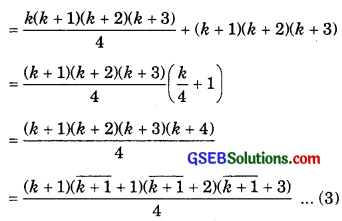∴ P(n) is true for n = k + 1, i.e., P(k + 1) is true.
∴ By Principle of Mathematical Induction, P(n) is true for all natural numbers n.

5. Let P(n) : 1.3 + 2.32 + 3.33 + … + n.3n = $$\frac{(2 n-1) \cdot 3^{n+1}+3}{4}$$
Putting n = 1, L.H.S.= 1.3 = 3
and R.H.S. = $$\frac{(2-1) \cdot 3^{2}+3}{4}$$ = $$\frac{12}{4}$$ = 3.
∴ L.H.S. = R.H.S.
This shows that P(n) is true for n = 1.
Let P(n) be true for n = k.
∴ P(k) : 1.3 + 2.32 + 3.32 + ………… + k.3k = $$\frac{(12 k-1) \cdot 3^{k+1}+3}{4}$$ is true. ………………. (1)
Adding (k + 1).3k+1 to both sides of (1), we get
L.H.S. = 1.3 + 2.32 + k.32 + … + k.3k + (k + 1).3k+1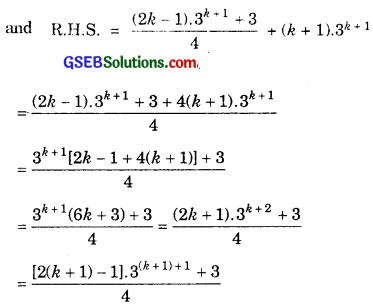This shows P(n) is true for n = k + 1.
i.e., P(k + 1) is true, whenever P(k) is true.
Hence, by principle of mathematical induction P(n) is true for all values of n ∈ N.6. Let P(n) : 1.2 + 2.3 + 3.4 + ………….. + n(n + 1) = $$\frac{n(n+1)(n+2)}{3}$$
For n = 1, L.H.S. = 1.2 = 2
and R.H.S. = $$\frac{n(n + 1)(n + 2)}{3}$$ = $$\frac{1.2.3}{3}$$ = 1.2 = 2.
i.e., L.H.S. = R.H.S.
So, P(1) is true.
We assume that P(n) is true for n = k.
i.e., 1.2 + 2.3 + 3.4 + … + k(k + 1) = $$\frac{k(k + 1)(k + 2)}{3}$$
Last term in L.H.S. is k(k + 1)
Replacing k by k + 1, we get (k + 1)(k + 2)
Adding it to both sides, we get
L.H.S. = 1.2 + 2.3 + 3.4 + … + k(k + 1) + (k + 1)(k + 2)Thus, P(k + 1) is true, whenever P(k) is true.
Hence, by principle of mathematical induction, P(n) is true for all values of n ∈ N.

7. Let P(n) be the given statement.
i.e., P(n) = 1.3 + 3.5 + 5.7 + … + (2n – 1)(2n + 1)
= $$\frac{n\left(4 n^{2}+6 n-1\right)}{3}$$
Putting n = 1,
L.H.S. = 1.3 = 3
and R.H.S. = $$\frac{1 .\left(4.1^{2}+6.1-1\right)}{3}$$ = $$\frac{4+6-1}{3}$$ = $$\frac{9}{3}$$ = 3.
L.H.S. = R.H.S.
∴ P(n) is true for n = 1.
Assume that P(n) is true for n = k, i.e., P(k) is true.
i.e., 1.3 + 3.5 + 3.7 + … + (2k – 1)(2k + 1) =$$\frac{k\left(4 k^{2}+6 k-1\right)}{3}$$
Last term in L.H.S. = (2k – 1)(2k + 1)
Replacing k by k + 1, we get
[2(k + 1) – 1][2(k + 1) + 1] = (2k + 1)(2k + 3)
Adding (2k + 1)(2k + 3) to both sides, we get
∴ L.H.S. = 1.3 + 3.5 + 5.7 + … + (2k – 1)(2k + 1) + (2k + 1)(2k + 3)Thus, P(n) is true for n = k + 1.
∴ P(k + 1) is true, whenever P(k) is true.
Hence, by principle of mathematical induction, P(n) is true for all n ∈ N.8. Let P(n) be the given statement.
i.e., P(n) : 1.2 + 2.22 + 3.23 + … + n.2n = (n – 1)2n+1 + 2
Putting n = 1, L.H.S. = 1.2 = 2
and R.H.S. = 0 + 2 = 2.
∴ P(n) is true for n = 1.
Assume that P(n) is true for n = k, i.e., P(k) is true, i.e.,
1.2 + 2.3 + 3.4 + … + k.2k = (k – 1).2k+1 + 2
Last term in L.H.S. = k.2k.
Replacing k by k + 1, we get the next term = (k + 1).2k+1
Adding it to both sides, we get
L.H.S. = 1.2 + 2.22 + 3.23 + … + k.2k+1 + 1 + (k + 1).2k+1
and R.H.S. = (k – 1).2k+1 + 2 + (k + 1).2k+1
= 2k+1.[k – 1 + k + 1] + 2 = 2k.2k+1 + 2
= k.2k+2 + 2.
This proves P(n) is true for n = k + 1.
Thus, P(k + 1) is true, whenever P(k) is true.
∴ By principle of mathematical induction, P(k) is true for all n ∈ N.9. Let P(n) be the given statement.
i.e., P(n): $$\frac{1}{2}$$ + $$\frac{1}{4}$$ + $$\frac{1}{8}$$ + ………….. + $$\frac{1}{2^{n}}$$ = 1 – $$\frac{1}{2^{n}}$$.
Putting n = 1, L.H.S. = $$\frac{1}{2}$$
and R.H.S. = 1 – $$\frac{1}{2}$$ = $$\frac{1}{2}$$.
∴ P(n) is true for n = 1.
Suppose P(n) is true for n = k.
∴ $$\frac{1}{2}$$ + $$\frac{1}{4}$$ + $$\frac{1}{8}$$ + …………… + $$\frac{1}{2^{k}}$$ = 1 – $$\frac{1}{2^{k}}$$
Last term in L.H.S. = $$\frac{1}{2^{k}}$$.
Replacing k by k + 1,
last term becomes $$\frac{1}{2^{k+1}}$$.
Adding $$\frac{1}{2^{k+1}}$$ to both sides, we get
L.H.S. = $$\frac{1}{2}$$ + $$\frac{1}{4}$$ + $$\frac{1}{8}$$ + ……………… + $$\frac{1}{2^{k}}$$ + $$\frac{1}{2^{k+1}}$$
and R.H.S. = 1 – $$\frac{1}{2^{k}}$$ + $$\frac{1}{2^{k+1}}$$ = 1 – $$\frac{1}{2^{k}}$$ (1 – $$\frac{1}{2}$$)
= 1 – $$\frac{1}{2^{k}}$$.$$\frac{1}{2}$$ = 1 – $$\frac{1}{2^{k+1}}$$.
This shows P(n) is true for n = k + 1.
Thus, P(k + 1) is true, whenever P(k) is true.
Thus, by principle of mathematical induction, P(n) is true for all n ∈N.

10. Let the given statement be P(n) i.e.,
P(n) : $$\frac{1}{2.5}$$ + $$\frac{1}{5.8}$$ + $$\frac{1}{8.11}$$ + ………….. + $$\frac{1}{(3n – 1)(3n + 2)}$$ = $$\frac{n}{6n + 4}$$.
Putting n = 1, L.H.S. = $$\frac{1}{2.5}$$ = $$\frac{1}{10}$$
and R.H.S. = $$\frac{1}{6 + 4}$$ = $$\frac{1}{10}$$.
∴ P(n) is true for n = 1.
Assuming P(n) is true for n = k, i.e., P(k) is true, i.e.,
$$\frac{1}{2.5}$$ + $$\frac{1}{3.8}$$ + $$\frac{1}{8.11}$$ + ………… + $$\frac{1}{(3k – 1)(3k + 1)}$$ = $$\frac{k}{6k + 4}$$.
Now, kth term = $$\frac{1}{(3k – 1)(3k + 2)}$$.
∴ (k + 1)th term = $$\frac{1}{[3(k + 1) – 1][3(k + 1) + 1]}$$ = $$\frac{1}{(3k + 2)(3k + 5)}$$.
Adding this term to both sides, we get
L.H.S. = $$\frac{1}{2.5}$$ + $$\frac{1}{3.8}$$ + $$\frac{1}{8.11}$$ + ………………. + $$\frac{1}{(3k – 1)(3k + 2)}$$ + $$\frac{1}{(3k + 2)(3k + 5)}$$
and R.H.S.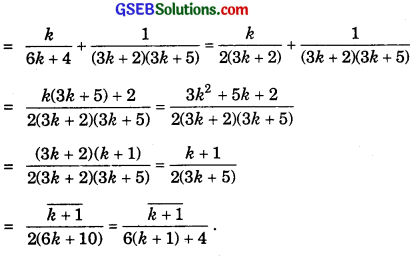This shows that P(n) is true for n = k + 1.
∴ P(k + 1) is true, whenever P(k) is true. So, by principle of mathematical induction, P(n) is true for all n ∈ N.11. Let P(n) be the given statement, i.e.,
P(n) : $$\frac{1}{1.2.3}$$ + $$\frac{1}{2.3.4}$$ + $$\frac{1}{3.4.5}$$ + ………….. + $$\frac{1}{n(n + 1) (n + 2)}$$ = $$\frac{n(n + 3)}{4(n + 1)(n + 2)}$$.
Putting n = 1, L.H.S. = $$\frac{1}{1.2.3}$$ = $$\frac{1}{6}$$
and R.H.S = $$\frac{1(1 + 3)}{4(1 + 1)(1 + 2)}$$ = $$\frac{4}{4.2.3}$$ = $$\frac{1}{6}$$.
∴ P(n) is true for n = 1.
Assuming P(n) is true for n = k, i.e., P(k) is supposed to be trueAdding this term to both sides, we get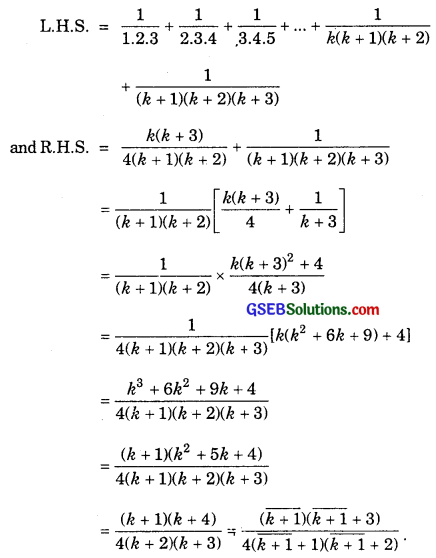This shows P(n) is true for n = k + 1, i.e., P(k + 1) is true whenever P(k) is true.
Hence, by principle of mathematical induction, P(n) is true for all n ∈ N.

12. Let P(n) : a + ar + ar2 + … + arn-1 = $$\frac{a\left(1-r^{n}\right)}{1-r}$$, r ≠ 1 ………………. (1)
For n = 1, L.H.S. = a
and R.H.S. = $$\frac{a(1-r)}{1-r}$$ = a.
∴ L.H.S. = R.H.S., i.e., P(1) is true.
Let us suppose that P(k) is true.
Putting n = k in (1), we have:
a + ar + ar2 + … + arn-1 = $$\frac{a\left(1-r^{n}\right)}{1-r}$$ …………….. (2)
Adding ark to both sides of (2), we have:
a + ar + ar2 + ……………… + ark-1 + ark∴ P(n) is true for n = k + 1, i.e., P(k + 1) is true.
Thus, P(k + 1) is true, whenever P(&) is true.
∴ By principle of mathematical induction, P(n) is true for all natural numbers n.

13. Let the given statement be denoted by P(n), i.e.,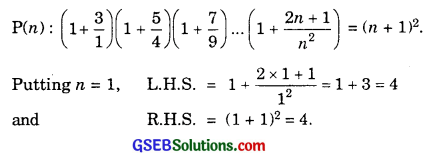L.H.S. = R.H.S.
∴ P(n) is true for n = 1.
Suppose P(k) is true.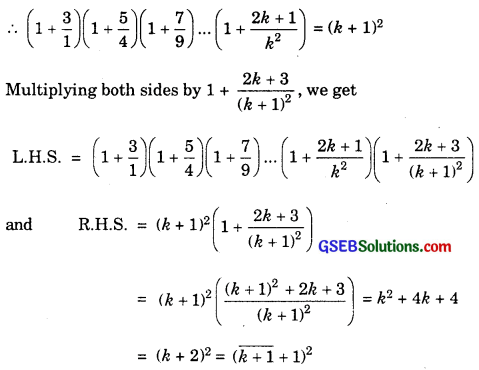∴ P(n) is true for n = k + 1, i.e., P(k + 1) is true, whenever P(k) is true.
Hence, by principle of mathematical induction, P(n) is true for all n ∈ N.14. Let the given statement be P(n), i.e.,
P(n) : (1 + $$\frac{1}{1}$$)(1 + $$\frac{1}{2}$$)(1 + $$\frac{1}{3}$$) ……………. (1 + $$\frac{1}{n}$$) = n + 1.
For n = 1, L.H.S. = 1 + $$\frac{1}{1}$$ = 2
and R.H.S. = 1 + 1 = 2.
∴ P(n) is true for n = 1.
Let P(&) be true, i.e.,
(1 + $$\frac{1}{1}$$)(1 + $$\frac{1}{2}$$)(1 + $$\frac{1}{3}$$) …………… (1 + $$\frac{1}{k}$$) = k + 1 is true.
Multiplying both sides by (1 + $$\frac{1}{k+1}$$), we get
L.H.S. = (1 + $$\frac{1}{1}$$)(1 + $$\frac{1}{2}$$)(1 + $$\frac{1}{3}$$) ………………. (1 + $$\frac{1}{k}$$)(1 + $$\frac{1}{k+1)}$$
and R.H.S = (k + 1) (1 + $$\frac{1}{k + 1}$$ = (k + 1)($$\frac{k+1+1)}{k+1}$$ = (k + 2)
= ($$\sqrt{k+1}$$ + 1)
Therefore P(k + 1) is also true, whenever P(k) is true. Hence, by principle of mathematical induction, P(n) is true for all n ∈ N.

15. Let the given statement be P(n), i.e.,
P(n) : 12 + 32 + 52 + … + (2n – 1)2 = $$\frac{n(2n – 1)(2n + 1)}{3}$$.
For n = 1, L.H.S. = 12 = 1
and R.H.S. = $$\frac{1.(2 – 1)(2 + 1)}{3}$$ = $$\frac{1.1.3}{3}$$ = 1.
∴ P(n) is true for n – 1.
Suppose P(&) is true for n = k i.e.,
12 + 32 + 52 + …………… + (2k – 1)2 = $$\frac{k(2k – 1)(2k + 1)}{3}$$.
Adding (2k + 1)2 to both sides, we get
L.H.S. = 12 + 32 + 52 + ……………. + (2k – 1)2 + (2k + 1)2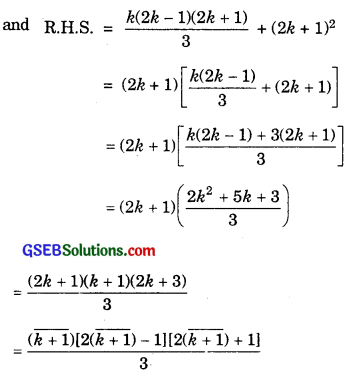Thus, P(k + 1) is true.
∴ P(k + 1) is true, whenever P(k) is true.
∴ By principle of mathematical induction, Pin) is true for all values of n ∈ N.16. Let the given statement be P(n), i.e.,
P(n) : $$\frac{1}{1.4}$$ + $$\frac{1}{4.7}$$ + $$\frac{1}{7.10}$$ + …………….. + $$\frac{1}{(3n – 2)(3n + 1)}$$ = $$\frac{n}{3n + 1}$$.
For n = 1, L.H.S. = $$\frac{1}{1.4}$$ = $$\frac{1}{4}$$
and R.H.S. = $$\frac{1}{3.1+1}$$ = $$\frac{1}{4}$$.
∴ P(n) is true for n = 1.
Assuming P(k) is true for some value of n = k.
∴ $$\frac{1}{1.4}$$ + $$\frac{1}{4.7}$$ + $$\frac{1}{7.10}$$ + ………………. + $$\frac{1}{(3k – 2)(3k + 1)}$$ = $$\frac{k}{3k + 1}$$.
Adding $$\frac{1}{(3k + 1)(3k + 2)}$$ both sides, we get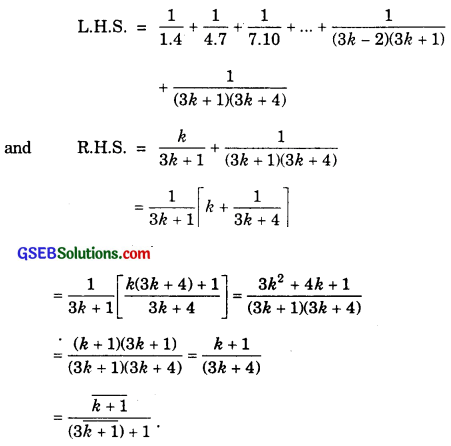Therefore, P(k + 1) is true for n = k + 1, i.e., P(k + 1) is true, whenever P(k) is true. Hence, by principle of mathematical induction, P(n) is true for all n ∈ N.

17. Let P(n) be the given statement, i.e.,
P(n) : $$\frac{1}{3.5}$$ + $$\frac{1}{5.7}$$ + $$\frac{1}{7.9}$$ + …………….. + $$\frac{1}{(2n + 1)(2n + 3)}$$ = $$\frac{n}{3(2n + 3)}$$.
For n = 1, L.H.S. = $$\frac{1}{3.5}$$ = $$\frac{1}{15}$$
and R.H.S. = $$\frac{1}{3.(2 + 3)}$$ = $$\frac{1}{3.5}$$ = $$\frac{1}{15}$$.
Thus, P(x) is true for n = 1.
Suppose P(k) be true for n = k, i.e.,
$$\frac{1}{3.5}$$ + $$\frac{1}{5.7}$$ + $$\frac{1}{7.9}$$ + ………….. + $$\frac{1}{(2k + 1)(2k + 3)}$$ = $$\frac{k}{3(2k + 3)}$$.
Adding $$\frac{1}{2(k + 3)(2k + 5)}$$ to both sides, we get
L.H.S. = $$\frac{1}{3.5}$$ + $$\frac{1}{5.7}$$ + $$\frac{1}{7.9}$$ + ……………. + $$\frac{1}{(2k + 1)(2k + 3)}$$ + $$\frac{1}{(2k + 3)(2k + 5)}$$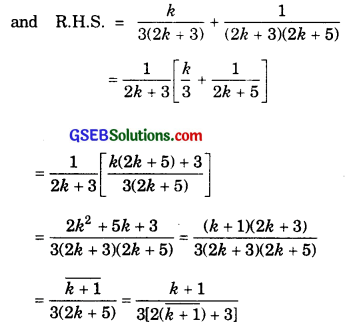Hence, P(k + 1) is true for n = k + 1, i.e., P(k + 1) is true, whenever P(k) is true.
Hence, by principle of mathematical induction, P(n) is true for all n ∈ N.18. Let P(n) : 1 + 2 + 3 + …………. + n < $$\frac{1}{8}$$ (2n + 1)2 ………………. (1)
For n = 1, (1) becomes 1 < $$\frac{1}{8}$$ (2 + 1)2 ⇒ 1 < $$\frac{9}{8}$$ ⇒ 1 < 1 $$\frac{1}{8}$$
which is true, i.e., P(1) is true.
Let us suppose that P(k) is true.
∴ Putting n = k in (1), we have:
1 + 2 + 3 + ………….. + k < $$\frac{1}{8}$$ (2k + 1)2
Adding (k + 1) to both sides of (2), we have:⇒ 1 + 2 + 3 + …………… + k + (k + 1) < $$\frac{1}{8}$$ (4k2 + 12k + 9)
⇒ 1 + 2 + 3 + ……………… + k + (k + 1) < $$\frac{1}{8}$$ (2k + 3)2
⇒ 1 + 2 + 3 + ……………. + k + (k + 1) < $$\frac{1}{8}$$ [2(k + 1) + 1]2 ……………… (3)
∴ P(k + 1) is true.
∴ By principle of mathematical induction, P(n) is true for all natural numbers n.

19. Let the statement be denoted by P(n), i.e.,
P(n) : n(n + 1)(n + 5) is a multiple of 3.
For n = 1, n(n + 1 )(n + 5) = 1.2.6 = 12 = 3.4.
P(n) is true for n = 1.
Suppose P(k) is true for n = k i.e.
k(k + 1)(k + 5) = 3m (say)
or k3 + 6k2 + 5k = 3m …………………… (1)
Replacing k by k + 1 in L.H.S. of (1), we get
(k + 1)(k + 2)(k + 6) = k(k2 + 8k + 12) + (k2 + 8k + 12)
= k3 + 9k2 + 20k + 12
= (k3 + 6k2 + 5k) + (3k2 – 15k + 12)
= 3m + 3k2 + 15k + 12 [From (1)]
= 3(m + k2 + 5k + 4).
i.e., (k + 1)(k + 2)(k + 6) is a multiple of 3, i.e.,
P(k + 1) is a multiple of 3, if P(k) is a multiple of 3.
i.e., P(k + 1) is true, whenever P(k) is true.
Hence, by principle of mathematical induction, P(n) is true for all n ∈ N.20. Let P(n) : 10(2n – 1) + 1 is divisible by 11 for every natural number n.
For n = 1, P(1) = 102-1 + 1 = 10 + 1 = 11,
which is divisible by 11
∴ P(1) is true.
Put n = k in (1), we have:
102k-1 + 1 is divisible by 11.
∴ 102k-1 + 1 = 11m, for some integer m.
⇒ 102k-1 = 11m – 1 …………………. (1)
Putting n = k + 1(k + 1) in 102n-1 + 1, it becomes
102(k+1)-1 + 1 = 102k+1 + 1 = 102k-1 = 102 + 1
= 100.102k-1 + 1 = 100(11m – 1) + 1 [Using (1)]
= 100 × 11m – 100 + 1
= 100 × 11m – 99
= 11(100m – 9), which is divisible by 11. [∵ 11 is a factor of R.H.S]
∴ 102(k+1)-1 + 1 is divisible by 11.
∴ P(n) is true for n = k + 1, i.e; P(k + 1) is true.
∴ By principle of mathematical induction, P(n) is true for all natural numbers n.

21. Let the statement be P(n), i.e.,
P (n) : x2n – y2n is divisible by x + y. ………………. (1)
Putting n = 1, x2n – y2n = x2 – y2 = (x + y)(x – y),
which is divisible by x + y.
⇒ P(n) is true for n = 1.
Let P(k) be true, i.e,
x2k – y2k is divisible by x + y
or x2k – y2k = m(x + y).
or x2k = m(x + y) + y2k ………………… (2)
Replace k by k + 1 in x2k from (2), we get
x2(k + 1) – y2(k + 1) = x2k+2 – y2k+2
= x2.x2k – y2k+2.
Putting the value of x2k from (2), we get
x2(k + 1) – y2(k + 1) = x2[m (x + y) + y2k] – y2k + 2
= m(x + y)x2 + x2y2k – y2k + 2
= m(x + y)2 + y2k)(x2 – y2)
= m(x + y)x2 + (x + y)(x – y)y2k
Therefore x2(k+1) – y2(k + 1) is divisible by (x + y).
i.e., P(k + 1) is true whenever P(k) is true.
By principle of mathematical induction, P(n) is true for all n ∈ N.22. Let the statement be denoted by P(n), i.e;
P(n) : 32n+2 – 8n – 9 is divisible by 8.
For n = 1, 32n+2 – 8n – 9 = 32+2 – 8.1 – 9
= 34 – 8 – 9 = 81 – 17 = 64,
which is divisible by 8.
Let P(k) be the true statement for some value of n = k.
∴ 32k+2 – 8k – 9 is divisible by 8.
or 32k+2 – 8k – 9 = 32(k + 1) + 2 – 8(k + 1) – 9
= 32k + 2 + 2 – 8k – 8 – 9
= 32. 32k+2 – 8k – 17
= 9.32k+2 – 8k – 17.
Putting the value of 32k+2 from (1), we get
22k+4 – 8k – 17 = 9(8m + 8k + 9) – 8k – 17
= 72m + 72k + 81 – 8k – 17
= 72m + 64k + 64
= 8(9m + 8k + 8)
Hence, 32k+4 – 8(k + 1) – 9 is divisible by 8.
i.e; P(k + 1) is true, whenever P(k) is true.
Hence, by principle of mathematical induction, P(n) is true for all n ∈ N.

23. Let P(n) be the given statement, i.e;
P(n) : 41n – 14n is a multiple of 27.
For n = 1, 41n – 14n = 41 – 14 = 27.
∴ P(n) is true for n = 1.
Let P(k) be true for some value of n = k.
P(k) : 41k – 14k = 27m, where m is a positive integer.
41k = 27 + 14k ……………… (1)
Replacing k by k + 1 in L.H.S. of (1), we get
41k + 1 – 14k + 1 = 41.41k – 14k + 1
= 41. (27m + 14k) – 14k + 1 [From (1)]
= 27.41 m + 14k(41 – 14)
= 27. 41m + 14k.27
= 27[41m + 14k]
This shows 41k + 1 – 14k + 1 is a multiple of 27.
or P(k + 1) is true, whenever P(k) is true.
Hence, by principal of mathematical induction, P(n) is true for all n ∈ N.24. Let P(n) : (2n + 7) < (n + 3)2 ………………. (1)
For n = 1, (1) becomes 2 × 1 + 7 < (1 + 3)2 ⇒ 9 < 16, which is true.
∴ P(1) is true
Let us suppose that P(k) is true.
∴ Putting n = k in (1), we have:
2(k + 7) < (k + 3)2 ………………….. (2)
Now, we will prove that P(k + 1) is true.
i.e; 2(k + 1) + 7 < (k + 4)2.
We know that 2k + 7 < (k + 3)2.
⇒ 2k + 7 + 2 < (k + 3)2 + 2 [Adding 2 t0 both sides]
⇒ 2(k + 1) + 7 < (k + 3)2 + 2 ……………… (3)
⇒ 2 (k + 1) + 7 < k2 + 6k + 11
Adding 2k + 5 to R.H.S., we get
⇒ 2(k + 1) + 7 < x2 + 7k + 16
or 2(k + 1) + 7 < (k + 4)2.
Thus, P(k + 1) is true. Hence, P(n) is true for n = k + 1.
∴ By principal of mathematical induction, P(n) is true for all natural numbers n.